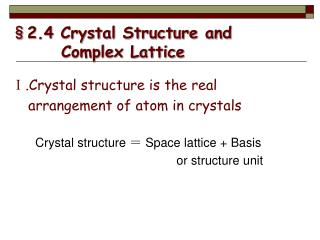DownloadDownload Presentation§2.4 Crystal Structure and Complex Lattice

# §2.4 Crystal Structure and Complex Lattice

Download Presentation## §2.4 Crystal Structure and Complex Lattice

- - - - - - - - - - - - - - - - - - - - - - - - - - - E N D - - - - - - - - - - - - - - - - - - - - - - - - - - -
##### Presentation Transcript

1. §2.4 Crystal Structure and Complex Lattice Ⅰ.Crystal structure is the real arrangement of atom in crystals Crystal structure ＝ Space lattice + Basis or structure unit

2. + =

3. Fe Al The difference between space lattice and crystal structure Fe : Al = 1 : 1

4. 2×3 atoms / cell

5. Ⅱ.Typical crystal structures of metals 1. BCC  Example: α-Fe , V, Nb, Ta, Cr, Mo, W, alkali metals  n =2 atoms/cell  CN=8  The number of nearest neighbours around each atom is called — Coordination Number.

6. Volume of atoms / cell Volume of unit cell  Packing fraction ＝ To determineξ, The atom is looked as a hard sphere, and the nearest neighbours touch each other. ∴ For BCC,

7. 2. FCC Example:γ-Fe , Al , Ni , Pb , Cu , Ag , Au , stainless steal  n= 8×1/8+6×1/2=4 atoms/cell  CN=12 

8. • • • • • • • • • • • • • • • •  Example:Be, Mg, Zn, Cd, Zr, Hf Ti( low temperature)  n＝  CN＝12  ξ＝0.74 3. HCP

9. 4. Summary

10. §2.5 Interstices in typical crystals of metals Definition: In any of the crystal structures, there are small holes between the usual atoms into which smaller atoms may be placed. These locations are called interstitial sites. Ⅰ. Two types of Interstitials in typical crystals Octahedral interstitial Tetrahedral interstitial

11. FCC

12. HCP

13. FCC

14. HCP

15. di da diameter of interstitial atom diameter of atom in lattice point ＝ Ⅱ.Determination of the sizes of interstitials Definition: By size of an interstitial we mean diameter of the maximum hard sphere which can be accommodated in the interstitial without distorting the lattice.

16. Octahedral interstitial condition for touching

17. For BCC For FCC

18. A B L interstitial • Tetrahedral interstitial H D host atom C

19. For BCC For FCC

20. Summary

21. Examples and Discussions • Both FCC and BCC are close-packed structures while BCC is more open? • The interstitial atoms most likely occupy the oct. interstitial position in FCC and HCP, while in BCC two types of interstitial can be occupied equally.

22. 3. The solid solubility in BCC is much lower than in FCC. 4. Diffusion of interstitial atoms in BCC diffusion is much faster than in FCC or HCP at same temperature. 5. Determine the relationship between the atomic radius and the lattice parameter in SC, BCC, and FCC structures when one atom is located at each lattice point.

23. 6. Determine the density of BCC iron, which has a lattice parameter of 0.2866nm. Solution: For a BCC cell, Atoms/cell = 2 a0 = 0.2866nm = 2.866×10－8cm Atomic mass = 55.847g/mol Volume of unit cell = a03 = 23.54×10 －24cm3/cell Density

24. c b a 120o Exercise • Determine the coordinates of centers of both the octahedral and the tetrahedral interstitials in HCP referred to a, b and c.

25. 4. Prove that the A-face-centered hexagonal lattice is not a new type of lattice in addition to the 14 space lattices. 5. Draw a primitive cell for BCC lattice. Thanks for your attention !